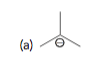Organic Chemistry Practice Problems Acid Base Equilibrium Practice Problems Solution: Write an equation for the proton transfer reaction...

# Solution: Write an equation for the proton transfer reaction that  occurs when each of the following bases reacts with water. In each case, draw curved arrows that show the mechanism of the proton transfer:

###### Problem

Write an equation for the proton transfer reaction that  occurs when each of the following bases reacts with water. In each case, draw curved arrows that show the mechanism of the proton transfer:Acid Base Equilibrium

Acid Base Equilibrium

#### Q. Write an equation for the proton transfer reaction that  occurs when each of the following bases reacts with water. In each case, draw curved arrows t...

Solved • Mon May 23 2016 17:16:57 GMT-0400 (EDT)

Acid Base Equilibrium

#### Q. Write an equation for the proton transfer reaction that  occurs when each of the following bases reacts with water. In each case, draw curved arrows t...

Solved • Mon May 23 2016 17:13:40 GMT-0400 (EDT)

Acid Base Equilibrium

#### Q. Write an equation for the proton transfer reaction that  occurs when each of the following bases reacts with water. In each case, draw curved arrows t...

Solved • Mon May 23 2016 17:09:24 GMT-0400 (EDT)

Acid Base Equilibrium

#### Q. Write an equation for the proton transfer reaction that occurs when each of the following acids reacts with water. In each case, draw curved arrows th...

Solved • Mon May 23 2016 16:41:02 GMT-0400 (EDT)